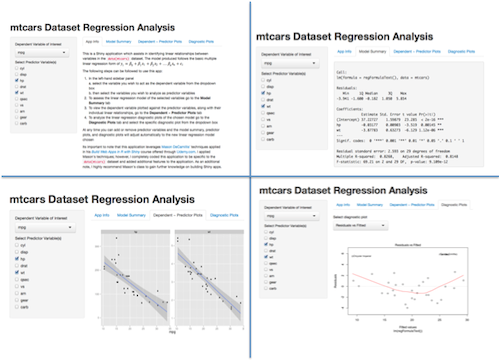# mtcars Dataset Regression Analysis

Brad Boehmke

## Synopsis

This Shiny application assists in identifying linear relationships between variables in the data(mtcars) dataset. The model produced follows the basic multiple linear regression form of $y_i = \beta_0 + \beta_1 x_1 + \beta_2 x_2 + ... \beta_n x_n + \epsilon_i$

### Why Do You Care?

1. When you have a dataset that contains multiple variables it can become tedious to perform initial analyses to identify linear relationships across multiple variables and to perform diagnostics. This app allows the user to quickly perform and analyze the performance of regression models in a dataset

2. The application proved to be quite useful for the Regression Models course project in which linear regression analysis was performed on this dataset

## How Do You Use It?

### The following steps can be followed to use this app:

1. In the left-hand sidebar panel
a. select the variable you wish to act as the dependent variable from the dropdown box
b. then select the variables you wish to analyze as predictor variables
2. To assess the linear regression model of the selected variables go to the Model Summary tab
3. To view the dependent variable plotted against the predictor variables, along with their individual linear relationships, go to the Dependent ~ Predictor Plots tab
4. To analyze the linear regression diagnostic plots of the chosen model go to the Diagnostic Plots tab and select the specific diagnostic plot from the dropdown box

At any time you can add or remove predictor variables and the model summary, predictor plots, and diagnostic plots will adjust automatically to the new linear regression model chosen

## Simplified Code Example

Although there is too much code to explain in this presentation, the primary objective of the application is to assess the performance of multiple regression within the mtcars dataset. The code used to perform the Regression models applies the basic lm() function to the selected variables. For example, if the variable mpg was selected to be regressed against hp and wt the following code will be produced along with the model summary:

lm(mpg ~ hp + wt, data = mtcars)

##
## Call:
## lm(formula = mpg ~ hp + wt, data = mtcars)
##
## Residuals:
##    Min     1Q Median     3Q    Max
## -3.941 -1.600 -0.182  1.050  5.854
##
## Coefficients:
##             Estimate Std. Error t value Pr(>|t|)
## (Intercept) 37.22727    1.59879   23.28  < 2e-16 ***
## hp          -0.03177    0.00903   -3.52   0.0015 **
## wt          -3.87783    0.63273   -6.13  1.1e-06 ***
## ---
## Signif. codes:  0 '***' 0.001 '**' 0.01 '*' 0.05 '.' 0.1 ' ' 1
##
## Residual standard error: 2.59 on 29 degrees of freedom
## Multiple R-squared:  0.827,  Adjusted R-squared:  0.815
## F-statistic: 69.2 on 2 and 29 DF,  p-value: 9.11e-12


## Screen Shot of Outputs

This application generates four primary outputs as shown in the screen shot below:

1. App Info: explains how to use the app to the user
2. Model Summary: Produces regression summary as seen on previous slide
3. Dependent~Predictor Plots: Plots $y_i$ against each $x_i$
4. Diagnostic Plots: Provides the residual diagnostic plots provided in lm() function